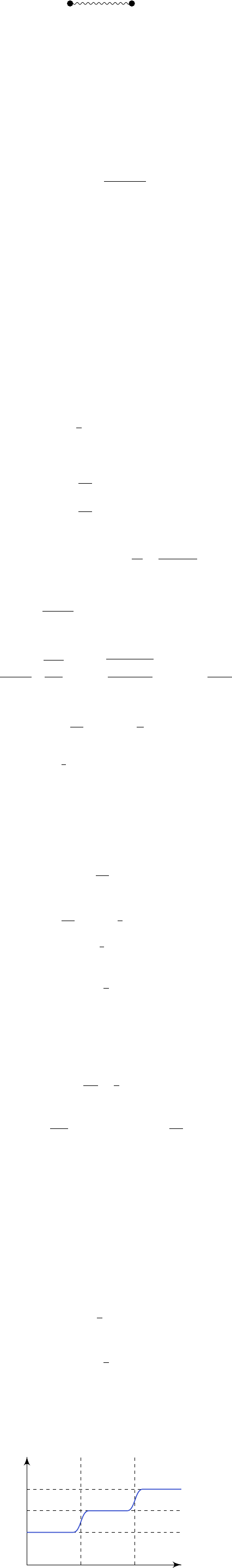2Classical gases

II Statistical Physics2.4 Diatomic gases
We now move on to consider more complicated gases. If we have molecules, then
they can contain other forms of energy such as rotational energy.
Everything we are doing is classical, so we need to come up with a model of a
diatomic molecule, instead of studying the actual quantum system of molecules.
For simplicity, we can model them as two point masses joined together by a
massless spring.
m
1
m
2
As we know from classical dynamics, we can decompose the motion into different
components:
Translation of the center of mass. We can view this as a single mass with
energy M = m
1
+ m
2
.
Rotations about center of mass. There are two axes of rotations orthogonal
to the spring, and these have a moment of inertia
I
. We will ignore the
rotation along the axes parallel to the spring because we assume the masses
are point masses.
Vibrations along the axis of symmetry. The important quantity here is the
reduced mass
m =
m
1
m
1
m
1
+ m
2
.
We will assume all these motions are independent. In reality, the translation is
indeed independent from the others, but the rotations and vibrations can couple
in complicated ways. But we are lazy, and will make this simplifying assumption,
we have
Z
1
= Z
trans
Z
rot
Z
vib
.
We can obtain
Z
trans
just as the partition energy we obtained previously for a
single mass, with mass
M
. The remaining two terms depend only on the relative
position of the masses. So they do not depend on the molecule as a whole, and
are going to be independent of V .
Now when we differentiate
log Z
1
with respect to
V
, then the latter two terms
drop out, and thus we deduce that the ideal gas law still holds for diatomic
gases.
We now try to figure out how rotations and vibrations contribute to the
partition function. For rotations, we can parametrize this using spherical polars
(θ, ϕ). The Lagrangian for this rotational motion is given by
L
rot
=
1
2
I(
˙
θ
2
+ sin
2
θ ˙ϕ
2
).
The conjugate momenta are given by
p
θ
=
L
˙
θ
= I
˙
θ
p
ϕ
=
L
˙ϕ
= I sin
2
θ ˙ϕ.
So we have
H
rot
=
˙
θp
θ
+ ˙ϕp
ϕ
L
rot
=
p
2
θ
2I
+
p
2
ϕ
2I sin
2
θ
.
We can then work out the partition function
Z
rot
=
1
(2π~)
2
Z
dθ dϕ dp
θ
dp
ϕ
e
βH
rot
We note that the p
θ
and p
ϕ
integrals are just Gaussians, and so we get
Z
rot
=
1
(2π~)
2
s
2πI
β
Z
π
0
dθ
s
2πI sin
2
θ
β
Z
2π
0
dϕ =
2IkT
~
2
.
Then we get
E
rot
=
β
log Z
rot
=
1
β
= kT.
This agrees with the equipartition of energy, as here we have two degrees of
freedom, and each contributes
1
2
kT .
Example.
In the case of a system where vibrations are not important, e.g. if
the string is very rigid, then we are done, and we have found
Z = Z
trans
Z
rot
(kT )
5/2
.
Then the partition function for N particles is
Z =
1
N!
Z
N
1
.
and the total energy is
β
log Z =
5
2
NkT.
This is exactly as we expect. There is
3
2
NkT
from translation, and
NkT
from
rotation. From this, we obtain the heat capacity
C
V
=
5
2
Nk.
We now put in the vibrations. Since we are modelling it by a spring, we
can treat it as a harmonic oscillator, with mass
m
and frequency
ω
, which is
determined by the bond strength. Then if we let
ζ
be the displacement form
equilibrium, then the Hamiltonian is
H
vib
=
p
2
ζ
2m
+
1
2
2
ζ
2
.
So we have
Z
vib
=
1
2π~
Z
dζ dp
ζ
e
βH
vib
=
kT
~ω
.
From the partition function, we can get the energy of a single molecule, and find
it to be
E
vib
= kT.
This is the average energy in the vibrational motion of a molecule. This looks a
bit funny. The vibration is only one degree of freedom, but the equipartition of
energy seems to think this has two degrees of freedom. It turns out equipartition
of energy behaves differently when we have potential energy. In general, we
should think of having one degree of freedom for each quadratic term in the
Hamiltonian, and so we have one degree of freedom for kinetic energy and another
for potential.
Putting all three types of motion together, we get
E =
7
2
NkT,
and the heat capacity is
C
V
=
7
2
Nk.
Note that these results are completely independent of the parameters describing
the molecule!
Does this agree with experiments? The answer is no! If we go and measure
the heat capacity of, say, molecular hydrogen, we find something like
T
C
V
/Nk
200 2000
1.5
2.5
3.5
So it seems like our prediction only works when we have high enough temperature.
At lower temperatures, the vibration modes freeze out. Then as we further lower
the energy, rotation modes also go away. This is a big puzzle classically! This is
explained by quantum effects, which we will discuss later.